# Mathematics - Discrete Mathematics - Binary Operations

in STEMGeeks11 months ago[Image 1]

## Introduction

Hey it's a me again @drifter1!

Today we continue with Mathematics, and more specifically the branch of "Discrete Mathematics", in order to get into Binary Operations.

So, without further ado, let's get straight into it!

## Binary Operations

For a non-empty set A a binary operation is a function of the form f : A x A → A. The two domains and the co-domain are the same exact set A. So, basically a binary operation is any rule that combines two elements (operands) in order to produce another element. For example, the arithmetic operations of addition, subtraction and multiplication are all binary operations on the set of integers.

The same logic can also be extended towards vector addition and matrix multiplication, by considering so called n-ary operations of the form f : A x A x ... x A → A.

Any binary operation can also be represented by a table of all possible combinations and their corresponding results. Considering the set of natural numbers, the binary operation of addition "+" can be represented by the table: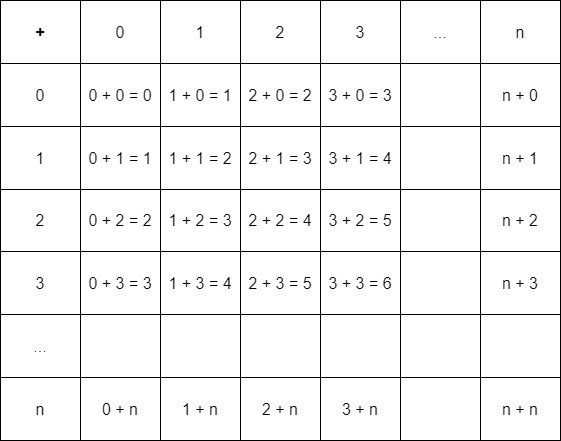## Properties

Binary operations may also satisfy lots of properties...

### Closure

For a non-empty set A and binary operation "*" on A, the binary operation satisfies closure when the result of the binary operation a * b ∈ A. Of course a, b ∈ A. For example, addition on the set of integers is a closed operation.

### Associativity

For a non-empty set A and binary operation "*" on A, the binary operation satisfies associativity if for every a, b, c ∈ A: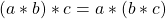### Commutativity

For a non-empty set A and binary operation "*" on A, the binary operation satisfies commutativity if for every a, b ∈ A, a * b = b * a.

### Distributivity

For a non-empty set A and binary operation "*" on A, the binary operation satisfies distributivity if for every a, b, c ∈ A: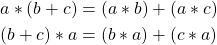which are the left and right distributivity respectively.

### Identity

For a non-empty set A and binary operation "*" on A, the binary operation satisfies the identity property if for every a ∈ A there exists an element e ∈ A, so that: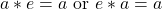which are known as the right and left identities respectively.

### Inverse

For a non-empty set A and binary operation "*" on A, the binary operation satisfies the inverse property if for every a ∈ A there exists an element b ∈ A, so that: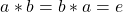which are known as the right and left inverse respectively.

### Idempotent

For a non-empty set A and binary operation "*" on A, the binary operation satisfies the idempotent property if for every a ∈ A : a * a = a.

### Cancellation

For a non-empty set A and binary operation "*" on A, the binary operation satisfies the cancellation property if for every a, b, c ∈ A: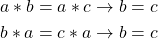which are the left and right cancellation respectively.

## RESOURCES:

### Images

Block diagrams and other visualizations were made using draw.io.

## Previous articles of the series

• Introduction → Discrete Mathematics, Why Discrete Math, Series Outline
• Sets → Set Theory, Sets (Representation, Common Notations, Cardinality, Types)
• Set Operations → Venn Diagrams, Set Operations, Properties and Laws
• Sets and Relations → Cartesian Product of Sets, Relation and Function Terminology (Domain, Co-Domain and Range, Types and Properties)
• Relation Closures → Relation Closures (Reflexive, Symmetric, Transitive), Full-On Example
• Equivalence Relations → Equivalence Relations (Properties, Equivalent Elements, Equivalence Classes, Partitions)
• Partial Order Relations and Sets → Partial Order Relations, POSET (Elements, Max-Min, Upper-Lower Bounds), Hasse Diagrams, Total Order Relations, Lattices
• Combinatorial Principles → Combinatorics, Basic Counting Principles (Additive, Multiplicative), Inclusion-Exclusion Principle (PIE)
• Combinations and Permutations → Factorial, Binomial Coefficient, Combination and Permutation (with / out repetition)
• Combinatorics Topics → Pigeonhole Principle, Pascal's Triangle and Binomial Theorem, Counting Derangements
• Propositions and Connectives → Propositional Logic, Propositions, Connectives (∧, ∨, →, ↔ and ¬)
• Implication and Equivalence Statements → Truth Tables, Implication, Equivalence, Propositional Algebra
• Proof Strategies (part 1) → Proofs, Direct Proof, Proof by Contrapositive, Proof by Contradiction
• Proof Strategies (part 2) → Proof by Cases, Proof by Counter-Example, Mathematical Induction
• Sequences and Recurrence Relations → Sequences (Terms, Definition, Arithmetic, Geometric), Recurrence Relations
• Probability → Probability Theory, Probability, Theorems, Example
• Conditional Probability → Conditional Probability, Law of Total Probability, Bayes' Theorem, Full-On Example
• Graphs → Graph Theory, Graphs (Vertices, Types, Handshake Lemma)
• Graphs 2 → Graph Representation (Adjacency Matrix and Lists), Graph Types and Properties (Isomorphic, Subgraphs, Bipartite, Regular, Planar)
• Paths and Circuits → Paths, Circuits, Euler, Hamilton
• Trees → Trees (Rooted, General and Binary), Tree Traversal, Spanning Trees
• Common Graph Problems → Shortest Path Problem, Graph Connectivity, Travelling Salesman Problem, Minimum Spanning Tree, Maximum Network Flow, Graph Coloring

## Final words | Next up

And this is actually it for today's post!

Next time we will start talking about Group Theory, where such binary operations are used.

See ya!Keep on drifting!

Posted with STEMGeeks

Sort:Happy Hive Birthday! You are on the Hive blockchain for 6 years!

Check out the last post from @hivebuzz: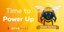Hive Power Up Day - May 1st 2022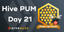Hive Power Up Month - Feedback from April day 21

You just reminded me of one of my favorite subjects in college - math. 🙂
Thank you for sharing.

!1UP#### You have received a 1UP from @thecuriousfool!

The following @oneup-cartel family members will soon upvote your post:
`@stem-curator`
And they will bring !PIZZA 🍕